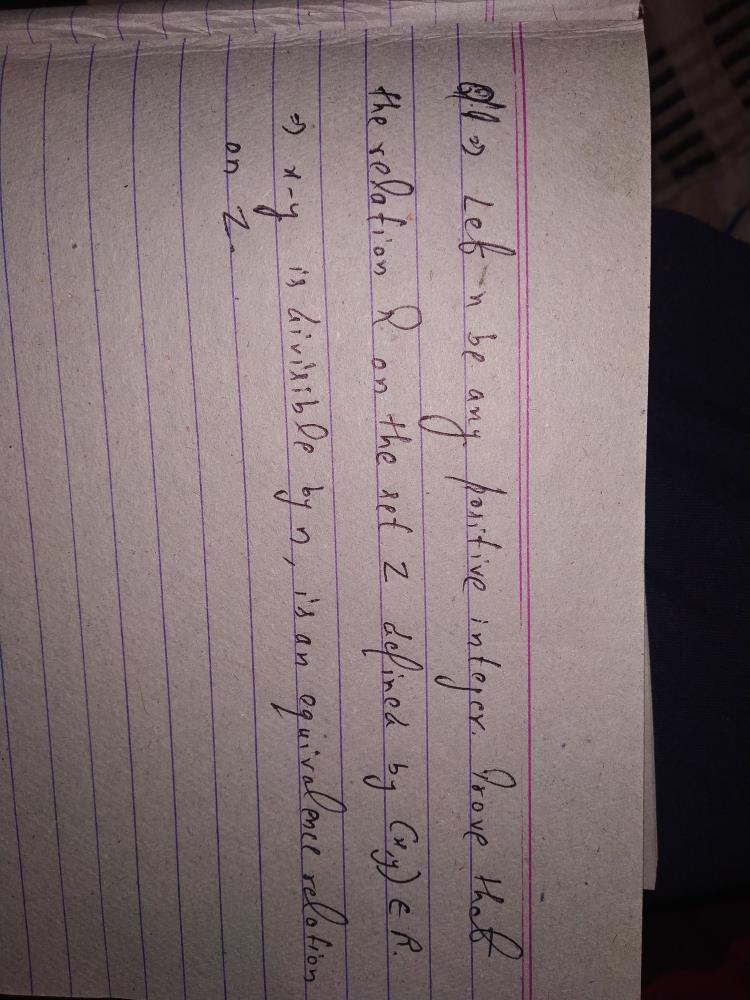Courses

# Intermediate (RELATIONS PART 1) Defence Notes | EduRev

## Defence : Intermediate (RELATIONS PART 1) Defence Notes | EduRev

The document Intermediate (RELATIONS PART 1) Defence Notes | EduRev is a part of Defence category.
All you need of Defence at this link: Defence
Here is the first part of relations.....
So What are these relations.???
It is the set containing all ordered pairs...where first element of that ordered pair belongs to first set and other belongs to the second one.... Now these elements are said to be in a relation...... Let us take an example...
Let A = (1,2,3).      and B = (3,4)
Now, A*B = [(1,3), (1,4), (2,3),(2,4),(3,3),(3,4)]
Let a relation be defined as...
R= [(1,3),(2,4)]
Now in this relation only element 1 and 2 of set A are related to 3 and 4 of set B.... In this way a relation is defined....
Also for a relation to be defined.. It must be a subset of the set A*B....

DOMAIN AND RANGE OF RELATIONS....                                                                                                                         Domain is the set of all first elements (which belongs to set A... and are in a relation with set B) In the above given example our domain will be
D= (1, 2) only.....
Talking about the range of a relation... It is the set of all second elements... Like in the example... Our range will be... (3,4) only....
One additional thing..... CODOMAIN OF A RELATION.       it is the set B on which alll elements of set A are related.... Range of a relation is always a subset of its CODOMAIN..... Now in the above example Codomain will be the set B.... i.e.(3,4).....As u can see range of relation i.e (3,4) is a subset of (3,4)....

TYPES OF RELATIONS.
1). IDENTITY RELATION - A Relation is said to be an identity relation if its every element is related to itself only.. . For a set A = [ 1,2]..identity relation be like [(1,1),(2,2)] BUT NOT [(1,1),(2,2),(1, 2)]

2)
REFLEXIVE RELATION - A relation is said to be reflexive if every element is related to itself(not only).... Let A be (1,2) reflexive relation be. [(1,1) (2,2)(1,2)]....

3)Symmetric relation - For all (a, b) belonging in relation... If (b, a) also belong in the relation then it is symmetric relation...

4) Transitive relation- For all (a, b) and (b, c) belonging in R.. If (a,c) also belongs to R then it is said to be transitive....

EQUIVALENCE RELATION
A relation is said to be equivalence relation if it is
1)Reflexive
2)Symmetric
3)transitive relation...Offer running on EduRev: Apply code STAYHOME200 to get INR 200 off on our premium plan EduRev Infinity!

,

,

,

,

,

,

,

,

,

,

,

,

,

,

,

,

,

,

,

,

,

;